# 73 Results

View
Selected filters:
• Calculus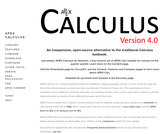Conditional Remix & Share Permitted
CC BY-NC
Rating

APEX Calculus is a calculus textbook written for traditional college/university calculus courses. It has the look and feel of the calculus book you likely use right now (Stewart, Thomas & Finney, etc.). The explanations of new concepts is clear, written for someone who does not yet know calculus. Each section ends with an exercise set with ample problems to practice & test skills (odd answers are in the back).

Access also available here: http://www.apexcalculus.com/

Chapter 1: Limits
Chapter 2: Derivatives
Chapter 3: The Graphical Behavior of Functions
Chapter 4: Applications of the Derivative
Chapter 5: Integration
Chapter 6: Techniques of Antidifferentiation
Chapter 7: Applications of Integration
Chapter 8: Sequences and Series
Chapter 9: Curves in the Plane
Chapter 10: Vectors
Chapter 11: Vector Valued Functions
Chapter 12: Functions of Several Variables
Chapter 13: Multiple Integrations
Chapter 14: Vector Analysis

Subject:
Calculus
Material Type:
Textbook
Provider:
APEX: Affordable Print and Electronic TeXtbooks
Author:
Brian Heinold
Dimplekumar Chalishajar
Gregory Hartman
Troy Siemers
01/01/2014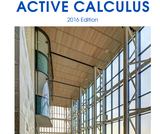Conditional Remix & Share Permitted
CC BY-NC-SA
Rating

Active Calculus is different from most existing calculus texts in at least the following ways: the text is free for download by students and instructors in .pdf format; in the electronic format, graphics are in full color and there are live html links to java applets; the text is open source, and interested instructors can gain access to the original source files upon request; the style of the text requires students to be active learners — there are very few worked examples in the text, with there instead being 3-4 activities per section that engage students in connecting ideas, solving problems, and developing understanding of key calculus concepts; each section begins with motivating questions, a brief introduction, and a preview activity, all of which are designed to be read and completed prior to class; the exercises are few in number and challenging in nature.

Subject:
Mathematics
Calculus
Material Type:
Textbook
Provider:
Grand Valley State University
Author:
David Austin
Matt Boelkins
Steve Schlicker
04/24/2019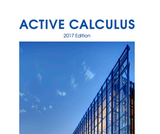Conditional Remix & Share Permitted
CC BY-NC-SA
Rating

Active Calculus is different from most existing calculus texts in at least the following ways: the text is freely readable online in HTML format and is also available for in PDF; in the electronic format, graphics are in full color and there are live links to java applets; version 2.0 now contains WeBWorK exercises in each chapter, which are fully interactive in the HTML format and included in print in the PDF; the text is open source, and interested users can gain access to the original source files on GitHub; the style of the text requires students to be active learners — there are very few worked examples in the text, with there instead being 3-4 activities per section that engage students in connecting ideas, solving problems, and developing understanding of key calculus concepts; each section begins with motivating questions, a brief introduction, and a preview activity, all of which are designed to be read and completed prior to class; following the WeBWorK exercises in each section, there are several challenging problems that require students to connect key ideas and write to communicate their understanding. For more information, see the author's website and blog.

Access also available here: https://scholarworks.gvsu.edu/books/15/

1 Understanding the Derivative
2 Computing Derivatives
3 Using Derivatives
4 The Definite Integral
5 Finding Antiderivatives and Evaluating Integrals
6 Using Definite Integrals
7 Differential Equations
8 Sequences and Series

Subject:
Calculus
Material Type:
Textbook
Author:
David Austin
Matthew Boelkins
Steven Schlicker
07/02/2019Conditional Remix & Share Permitted
CC BY-SA
Rating

Active Calculus is different from most existing calculus texts in at least the following ways: the text is freely readable online in HTML format and is also available for in PDF; in the electronic format, graphics are in full color and there are live links to java applets; there are live WeBWorK exercises in each chapter, which are fully interactive in the HTML format and included in print in the PDF; the text is open source, and interested users can gain access to the original source files on GitHub; the style of the text requires students to be active learners — there are very few worked examples in the text, with there instead being 3-4 activities per section that engage students in connecting ideas, solving problems, and developing understanding of key calculus concepts; each section begins with motivating questions, a brief introduction, and a preview activity, all of which are designed to be read and completed prior to class; following the WeBWorK exercises in each section, there are several challenging problems that require students to connect key ideas and write to communicate their understanding. For more information, see the author's website and blog.

Subject:
Calculus
Material Type:
Textbook
Author:
David Austin
Matthew Boelkins
Steven Schlicker
07/02/2019Conditional Remix & Share Permitted
CC BY-SA
Rating

2017 edition. Active Calculus Multivariable is the continuation of Active Calculus to multivariable functions. The Active Calculus texts are different from most existing calculus texts in at least the following ways: the texts are free for download by students and instructors in .pdf format; in the electronic format, graphics are in full color and there are live html links to java applets; the texts are open source, and interested instructors can gain access to the original source files upon request; the style of the texts requires students to be active learners — there are very few worked examples in the texts, with there instead being 3-4 activities per section that engage students in connecting ideas, solving problems, and developing understanding of key calculus concepts; each section begins with motivating questions, a brief introduction, and a preview activity, all of which are designed to be read and completed prior to class; the exercises are few in number and challenging in nature.

Access also available here: https://scholarworks.gvsu.edu/books/14/

Subject:
Calculus
Material Type:
Textbook
Author:
David Austin
Matthew Boelkins
Steven Schlicker
07/02/2019Conditional Remix & Share Permitted
CC BY-NC-SA
Rating

Active Calculus Multivariable is the continuation of Active Calculus to multivariable functions. The Active Calculus texts are different from most existing calculus texts in at least the following ways: the texts are freely readable online in HTML format (new in this version of Active Calculus Multivariable) and are also available for in PDF; in the electronic format, graphics are in full color; the texts are open source, and interested instructors can gain access to the original source files on GitHub; the style of the texts requires students to be active learners — there are very few worked examples in the texts, with there instead being 3-4 activities per section that engage students in connecting ideas, solving problems, and developing understanding of key calculus concepts; each section begins with motivating questions, a brief introduction, and a preview activity, all of which are designed to be read and completed prior to class; each section contains a collection of WeBWorK exercises (with solutions available in the HTML version, new in this version) followed by several challenging problems that require students to connect key ideas and write to communicate their understanding.

Subject:
Calculus
Material Type:
Textbook
Author:
David Austin
Matthew Boelkins
Steven Schlicker
07/02/2019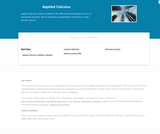Educational Use
Rating

Applied Calculus instructs students in the differential and integral calculus of elementary functions with an emphasis on applications to business, social and life science. Different from a traditional calculus course for engineering, science and math majors, this course does not use trigonometry, nor does it focus on mathematical proofs as an instructional method.

Subject:
Calculus
Material Type:
Full Course
Textbook
Provider:
Lumen Learning
Provider Set:
Candela Courseware
Author:
Dale Hoffman
David Lippman
Shana Calaway
04/25/2019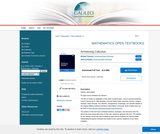Conditional Remix & Share Permitted
CC BY-NC-SA
Rating

The text is mostly an adaptation of two other excellent open- source calculus textbooks: Active Calculus by Dr. Matt Boelkins of Grand Valley State University and Drs. Gregory Hartman, Brian Heinold, Troy Siemers, Dimplekumar Chalishajar, and Jennifer Bowen of the Virginia Military Institute and Mount Saint Mary's University. Both of these texts can be found at http://aimath.org/textbooks/approved-textbooks/.
The authors of this text have combined sections, examples, and exercises from the above two texts along with some of their own content to generate this text. The impetus for the creation of this text was to adopt an open-source textbook for Calculus while maintaining the typical schedule and content of the calculus sequence at our home institution.

Subject:
Calculus
Material Type:
Textbook
Provider:
University System of Georgia
Provider Set:
Galileo Open Learning Materials
Author:
Jared Schlieper,
Michael Tiemeyer
03/20/2015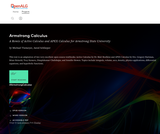Conditional Remix & Share Permitted
CC BY-NC-SA
Rating

This text is an adaptation of two very excellent open-source textbooks: Active Calculus by Dr. Matt Boelkins and APEX Calculus by Drs. Gregory Hartman, Brian Heinold, Troy Siemers, Dimplekumar Chalishajar, and Jennifer Bowen. Topics include integrals, volume, arcs, density, physics applications, differential equations, and hyperbolic functions.

1. Using Definite Integrals to Find Volume
2. Volume by The Shell Method
3. Arc Length and Surface Area
4. Density, Mass, and Center of Mass
5. Physics Applications: Work, Force, and Pressure
6. An Introduction to Differential Equations
7. Separable differential equations
8. Hyperbolic Functions

Subject:
Calculus
Material Type:
Textbook
Author:
Jared Schlieper
Michael Tiemeyer
01/23/2020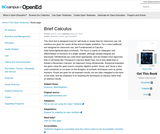Unrestricted Use
CC BY
Rating

This short text is designed more for self-study or review than for classroom use; full solutions are given for nearly all the end-of-chapter problems. For a more traditional text designed for classroom use, see Fundamentals of Calculus (http://www.lightandmatter.com/fund/). The focus is mainly on integration and differentiation of functions of a single variable, although iterated integrals are discussed. Infinitesimals are used when appropriate, and are treated more rigorously than in old books like Thompson's Calculus Made Easy, but in less detail than in Keisler's Elementary Calculus: An Approach Using Infinitesimals. Numerical examples are given using the open-source computer algebra system Yacas, and Yacas is also used sometimes to cut down on the drudgery of symbolic techniques such as partial fractions. Proofs are given for all important results, but are often relegated to the back of the book, and the emphasis is on teaching the techniques of calculus rather than on abstract results.

Subject:
Mathematics
Calculus
Material Type:
Textbook
Provider:
BCcampus
Provider Set:
BCcampus Open Textbooks
Author:
Benjamin Crowell
10/28/2014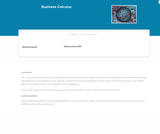Educational Use
Rating

This course provides an introduction to applied concepts in Calculus that are relevant to the managerial, life, and social sciences. Students should have a firm grasp of the concept of functions to succeed in this course. Topics covered include derivatives of basic functions and how they can be used to optimize quantities such as profit and revenues, as well as integrals of basic functions and how they can be used to describe the total change in a quantity over time.

Subject:
Calculus
Material Type:
Full Course
Textbook
Provider:
Lumen Learning
Provider Set:
Candela Courseware
04/25/2019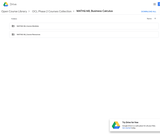Unrestricted Use
CC BY
Rating

MATH&148 is a calculus course for business students. It is designed for students who want a brief course in calculus. Topics include differential and integral calculus of elementary functions. Problems emphasize business and social science applications. Translating words into mathematics and solving word problems are emphasized over algebra. Applications are mainly business oriented (e.g. cost, revenue, and profit). Mathematical theory and complex algebraic manipulations are not mainstays of this course, which is designed to be less rigorous than the calculus sequence for scientists and engineers. Topics are presented according to the rule of four: geometrically, numerically, analytically, and verbally. That is, symbolic manipulation must be balanced with graphical interpretation, numerical examples, and writing. Trigonometry is not part of the course.

Subject:
Calculus
Material Type:
Assessment
Full Course
Syllabus
Textbook
Provider:
Washington State Board for Community & Technical Colleges
Provider Set:
Open Course Library
04/04/2019Conditional Remix & Share Permitted
CC BY-NC-SA
Rating

Published in 1991 by Wellesley-Cambridge Press, the book is a useful resource for educators and self-learners alike. It is well organized, covers single variable and multivariable calculus in depth, and is rich with applications.

In addition to the Textbook, there is also an online Instructor's Manual and a student Study Guide. Prof. Strang has also developed a related series of videos, Highlights of Calculus, on the basic ideas of calculus.

Subject:
Mathematics
Calculus
Material Type:
Full Course
Provider:
M.I.T.
Author:
Gilbert Strang
01/01/1991Conditional Remix & Share Permitted
CC BY-NC-SA
Rating

Published in 1991 and still in print from Wellesley-Cambridge Press, the book is a useful resource for educators and self-learners alike. It is well organized, covers single variable and multivariable calculus in depth, and is rich with applications. There is also an online Instructor's Manual and a student Study Guide. Prof. Strang has also developed a related series of videos, Highlights of Calculus, on the basic ideas of calculus.

Chapter 1: Introduction to Calculus
Chapter 2: Derivatives
Chapter 3: Applications of the Derivative
Chapter 4: The Chain Rule
Chapter 5: Integrals
Chapter 6: Exponentials and Logarithms
Chapter 7: Techniques of Integration
Chapter 8: Applications of the Integral
Chapter 9: Polar Coordinates and Complex Numbers
Chapter 10: Infinite Series
Chapter 11: Vectors and Matrices
Chapter 12: Motion along a Curve
Chapter 13: Partial Derivatives
Chapter 14: Multiple Integrals
Chapter 15: Vector Calculus
Chapter 16: Mathematics after Calculus

Also available from: https://ocw.mit.edu/resources/res-18-001-calculus-online-textbook-spring-2005/

Subject:
Calculus
Material Type:
Full Course
Provider:
M.I.T.
Provider Set:
M.I.T. OpenCourseWare
Author:
Gilbert Strang
01/01/2005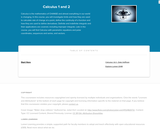Educational Use
Rating

Calculus is the mathematics of CHANGE and almost everything in our world is changing. In this course, you will investigate limits and how they are used to calculate rate of change at a point, define the continuity of a function and how they are used to define derivatives. Definite and indefinite integrals and their applications are covered, including improper integrals. Late in the course, you will find Calculus with parametric equations and polar coordinates, sequences and series, and vectors.

Subject:
Calculus
Material Type:
Full Course
Textbook
Provider:
Lumen Learning
Provider Set:
Candela Courseware
Author:
Dale Hoffman
David Lippman
Jeff Eldridge
Melonie Rasmussen
04/25/2019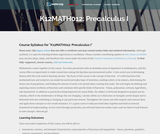Unrestricted Use
CC BY
Rating

This course is oriented toward US high school students. Its structure and materials are aligned to the US Common Core Standards. Foci include: derivatives, integrals, limits, approximation, and applications.

Subject:
Calculus
Material Type:
Full Course
Provider:
The Saylor Foundation
04/29/2019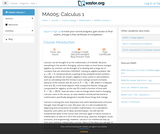Unrestricted Use
CC BY
Rating

This course begins with a review of algebra specifically designed to help and prepare the student for the study of calculus, and continues with discussion of functions, graphs, limits, continuity, and derivatives. The appendix provides a large collection of reference facts, geometry, and trigonometry that will assist in solving calculus problems long after the course is over. Upon successful completion of this course, the student will be able to: calculate or estimate limits of functions given by formulas, graphs, or tables by using properties of limits and LĺÎĺ_ĺĚĺ_hopitalĺÎĺ_ĺĚĺ_s Rule; state whether a function given by a graph or formula is continuous or differentiable at a given point or on a given interval and justify the answer; calculate average and instantaneous rates of change in context, and state the meaning and units of the derivative for functions given graphically; calculate derivatives of polynomial, rational, common transcendental functions, and implicitly defined functions; apply the ideas and techniques of derivatives to solve maximum and minimum problems and related rate problems, and calculate slopes and rates for function given as parametric equations; find extreme values of modeling functions given by formulas or graphs; predict, construct, and interpret the shapes of graphs; solve equations using NewtonĺÎĺ_ĺĚĺ_s Method; find linear approximations to functions using differentials; festate in words the meanings of the solutions to applied problems, attaching the appropriate units to an answer; state which parts of a mathematical statement are assumptions, such as hypotheses, and which parts are conclusions. This free course may be completed online at any time. It has been developed through a partnership with the Washington State Board for Community and Technical Colleges; the Saylor Foundation has modified some WSBCTC materials. (Mathematics 005)

Subject:
Calculus
Functions
Material Type:
Assessment
Full Course
Syllabus
Textbook
Provider:
The Saylor Foundation
04/29/2019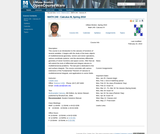Conditional Remix & Share Permitted
CC BY-NC-SA
Rating

This course is an introduction to the calculus of functions of several variables. It begins with studying the basic objects of multidimensional geometry: vectors and vector operations, lines, planes, cylinders, quadric surfaces, and various coordinate systems. It continues with the elementary differential geometry of vector functions and space curves. After this, it extends the basic tools of differential calculus - limits, continuity, derivatives, linearization, and optimization - to multidimensional problems. The course will conclude with a study of integration in higher dimensions, culminating in a multidimensional version of the substitution rule.

Subject:
Calculus
Geometry
Material Type:
Full Course
Homework/Assignment
Syllabus
Provider:
UMass Boston
Provider Set:
UMass Boston OpenCourseWare
Author:
Catalin Zara
Ph.D.
04/25/2019Unrestricted Use
CC BY
Rating

This contemporary calculus course is the third in a three-part sequence. In this course students continue to explore the concepts, applications, and techniques of Calculus - the mathematics of change. Calculus has wide-spread application in science, economics and engineering, and is a foundation college course for further work in these areas. This is a required class for most science and mathematics majors.Login: guest_oclPassword: ocl

Subject:
Calculus
Material Type:
Full Course
Homework/Assignment
Lecture Notes
Syllabus
Provider:
Washington State Board for Community & Technical Colleges
Provider Set:
Open Course Library
10/31/2011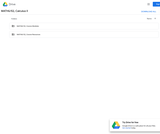Unrestricted Use
CC BY
Rating

This contemporary calculus course is the second in a three-part sequence. In this course students continue to explore the concepts, applications, and techniques of Calculus - the mathematics of change. Calculus has wide-spread application in science, economics and engineering, and is a foundation college course for further work in these areas. This is a required class for most science and mathematics majors.Login: guest_oclPassword: ocl

Subject:
Calculus
Material Type:
Full Course
Homework/Assignment
Lecture Notes
Syllabus
Provider:
Washington State Board for Community & Technical Colleges
Provider Set:
Open Course Library
10/31/2011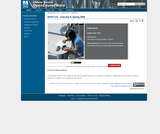Conditional Remix & Share Permitted
CC BY-NC-SA
Rating

Topics in this course include transcendental functions, techniques of integration, applications of the integral, improper integrals, l'Hospital's rule, sequences, and series.

Subject:
Calculus
Material Type:
Full Course
Homework/Assignment
Syllabus
Provider:
UMass Boston
Provider Set:
UMass Boston OpenCourseWare
Author:
Catalin Zara
Ph.D.
04/25/2019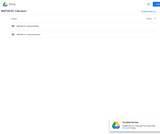Unrestricted Use
CC BY
Rating

This course is an introduction to contemporary calculus and is the first of a three-part sequence. In this course students explore the concepts, applications, and techniques of Calculus - the mathematics of change. Calculus has wide-spread application in science, economics and engineering, and is a foundation college course for further work in these areas. This is a required class for most science and mathematics majors.Login: guest_oclPassword: ocl

Subject:
Calculus
Functions
Material Type:
Full Course
Homework/Assignment
Lecture Notes
Syllabus
Provider:
Washington State Board for Community & Technical Colleges
Provider Set:
Open Course Library
10/31/2011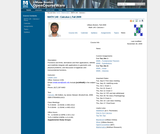Conditional Remix & Share Permitted
CC BY-NC-SA
Rating

This course is an introduction to differential and integral calculus. It begins with a short review of basic concepts surrounding the notion of a function. Then it introduces the important concept of the limit of a function, and use it to study continuity and the tangent problem. The solution to the tangent problem leads to the study of derivatives and their applications. Then it considers the area problem and its solution, the definite integral. The course concludes with the calculus of elementary transcendental functions.

Subject:
Calculus
Material Type:
Full Course
Homework/Assignment
Syllabus
Provider:
UMass Boston
Provider Set:
UMass Boston OpenCourseWare
Author:
Catalin Zara
04/25/2019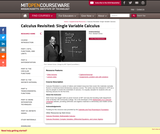Conditional Remix & Share Permitted
CC BY-NC-SA
Rating

Calculus Revisited is a series of videos and related resources that covers the materials normally found in a freshman-level introductory calculus course.&nbsp; The series was first released in 1970 as a way for people to review the essentials of calculus.&nbsp; It is equally valuable for students who are learning calculus for the first time.

Subject:
Calculus
Material Type:
Full Course
Provider:
M.I.T.
Provider Set:
M.I.T. OpenCourseWare
Author:
Gross, Herbert
11/18/2012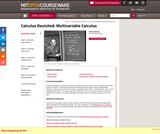Conditional Remix & Share Permitted
CC BY-NC-SA
Rating

Calculus Revisited is a series of videos and related resources that covers the materials normally found in freshman- and sophomore-level introductory mathematics courses. Multivariable Calculus is the second course in the series, consisting of 26 videos, 4 Study Guides, and a set of Supplementary Notes. The series was first released in 1971 as a way for people to review the essentials of calculus. It is equally valuable for students who are learning calculus for the first time.

Subject:
Calculus
Material Type:
Full Course
Provider:
M.I.T.
Provider Set:
M.I.T. OpenCourseWare
Author:
Herbert Gross
01/01/2011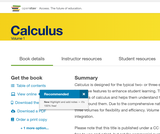Conditional Remix & Share Permitted
CC BY-NC-SA
Rating

Calculus is designed for the typical two- or three-semester general calculus course, incorporating innovative features to enhance student learning. The book guides students through the core concepts of calculus and helps them understand how those concepts apply to their lives and the world around them. Due to the comprehensive nature of the material, we are offering the book in three volumes for flexibility and efficiency. Volume 1 covers functions, limits, derivatives, and integration.

Chapter 1: Functions and Graphs
Chapter 2: Limits
Chapter 3: Derivatives
Chapter 4: Applications of Derivatives
Chapter 5: Integration
Chapter 6: Applications of Integrations

Also available here: https://openstax.org/details/books/calculus-volume-1

Subject:
Calculus
Material Type:
Textbook
Provider:
Rice University
Provider Set:
OpenStax College
Author:
Alfred K. Mulzet
Catherine Abbott
David McCune
David Smith
David Torain
Edwin Jed Herman
Elaine A. Terry
Erica M. Rutter
Gilbert Strang
Joseph Lakey
Joyati Debnath
Julie Levandosky
Kirsten R. Messer
Michelle Merriweather
Nicoleta Virginia Bila
Sheri J. Boyd
Valeree Falduto
02/01/2016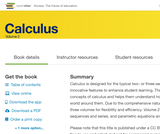Conditional Remix & Share Permitted
CC BY-NC-SA
Rating

Calculus is designed for the typical two- or three-semester general calculus course, incorporating innovative features to enhance student learning. The book guides students through the core concepts of calculus and helps them understand how those concepts apply to their lives and the world around them. Due to the comprehensive nature of the material, we are offering the book in three volumes for flexibility and efficiency. Volume 2 covers integration, differential equations, sequences and series, and parametric equations and polar coordinates.

Access also available here: https://openstax.org/details/books/calculus-volume-2

Chapter 1: Integration

1.1 Approximating Areas
1.2 The Definite Integral
1.3 The Fundamental Theorem of Calculus
1.4 Integration Formulas and the Net Change Theorem
1.5 Substitution
1.6 Integrals Involving Exponential and Logarithmic Functions
1.7 Integrals Resulting in Inverse Trigonometric Functions
Chapter 2: Applications of Integration

2.1 Areas between Curves
2.2 Determining Volumes by Slicing
2.3 Volumes of Revolution: Cylindrical Shells
2.4 Arc Length of a Curve and Surface Area
2.5 Physical Applications
2.6 Moments and Centers of Mass
2.7 Integrals, Exponential Functions, and Logarithms
2.8 Exponential Growth and Decay
2.9 Calculus of the Hyperbolic Functions
Chapter 3: Techniques of Integration

3.1 Integration by Parts
3.2 Trigonometric Integrals
3.3 Trigonometric Substitution
3.4 Partial Fractions
3.5 Other Strategies for Integration
3.6 Numerical Integration
3.7 Improper Integrals
Chapter 4: Introduction to Differential Equations

4.1 Basics of Differential Equations
4.2 Direction Fields and Numerical Methods
4.3 Separable Equations
4.4 The Logistic Equation
4.5 First-order Linear Equations
Chapter 5: Sequences and Series

5.1 Sequences
5.2 Infinite Series
5.3 The Divergence and Integral Tests
5.4 Comparison Tests
5.5 Alternating Series
5.6 Ratio and Root Tests
Chapter 6: Power Series

6.1 Power Series and Functions
6.2 Properties of Power Series
6.3 Taylor and Maclaurin Series
6.4 Working with Taylor Series
Chapter 7: Parametric Equations and Polar Coordinates

7.1 Parametric Equations
7.2 Calculus of Parametric Curves
7.3 Polar Coordinates
7.4 Area and Arc Length in Polar Coordinates
7.5 Conic Sections

Subject:
Calculus
Material Type:
Textbook
Provider:
Rice University
Provider Set:
OpenStax College
Author:
Alfred K. Mulzet
Catherine Abbott
David McCune
David Smith
David Torain
Edwin Jed Herman
Elaine A. Terry
Erica M. Rutter
Gilbert Strang
Joseph Lakey
Joyati Debnath
Julie Levandosky
Kirsten R. Messer
Michelle Merriweather
Nicoleta Virginia Bila
Sheri J. Boyd
Valeree Falduto
02/01/2016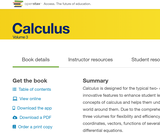Conditional Remix & Share Permitted
CC BY-NC-SA
Rating

Calculus is designed for the typical two- or three-semester general calculus course, incorporating innovative features to enhance student learning. The book guides students through the core concepts of calculus and helps them understand how those concepts apply to their lives and the world around them. Due to the comprehensive nature of the material, we are offering the book in three volumes for flexibility and efficiency. Volume 3 covers parametric equations and polar coordinates, vectors, functions of several variables, multiple integration, and second-order differential equations.

Access also available here: https://openstax.org/details/books/calculus-volume-3

1. Parametric Equations and Polar Coordinates

1.1. Introduction
1.2. Parametric Equations
1.3. Calculus of Parametric Curves
1.4. Polar Coordinates
1.5. Area and Arc Length in Polar Coordinates
1.6. Conic Sections
2. Vectors in Space

2.1. Introduction
2.2. Vectors in the Plane
2.3. Vectors in Three Dimensions
2.4. The Dot Product
2.5. The Cross Product
2.6. Equations of Lines and Planes in Space
2.8. Cylindrical and Spherical Coordinates
3. Vector-Valued Functions

3.1. Introduction
3.2. Vector-Valued Functions and Space Curves
3.3. Calculus of Vector-Valued Functions
3.4. Arc Length and Curvature
3.5. Motion in Space
4. Differentiation of Functions of Several Variables

4.1. Introduction
4.2. Functions of Several Variables
4.3. Limits and Continuity
4.4. Partial Derivatives
4.5. Tangent Planes and Linear Approximations
4.6. The Chain Rule
4.7. Directional Derivatives and the Gradient
4.8. Maxima/Minima Problems
4.9. Lagrange Multipliers
5. Multiple Integration

5.1. Introduction
5.2. Double Integrals over Rectangular Regions
5.3. Double Integrals over General Regions
5.4. Double Integrals in Polar Coordinates
5.5. Triple Integrals
5.6. Triple Integrals in Cylindrical and Spherical Coordinates
5.7. Calculating Centers of Mass and Moments of Inertia
5.8. Change of Variables in Multiple Integrals
6. Vector Calculus

6.1. Introduction
6.2. Vector Fields
6.3. Line Integrals
6.4. Conservative Vector Fields
6.5. Green's Theorem
6.6. Divergence and Curl
6.7. Surface Integrals
6.8. Stokes' Theorem
6.9. The Divergence Theorem
7. Second-Order Differential Equations

7.1. Introduction
7.2. Second-Order Linear Equations
7.3. Nonhomogeneous Linear Equations
7.4. Applications
7.5. Series Solutions of Differential Equations
Table of Integrals
Table of Derivatives
Review of Pre-Calculus

Subject:
Calculus
Material Type:
Textbook
Provider:
Rice University
Provider Set:
OpenStax College
Author:
Alfred K. Mulzet
Catherine Abbott
David McCune
David Smith
David Torain
Edwin Jed Herman
Elaine A. Terry
Erica M. Rutter
Gilbert Strang
Joseph Lakey
Joyati Debnath
Julie Levandosky
Kirsten R. Messer
Michelle Merriweather
Nicoleta Virginia Bila
Sheri J. Boyd
Valeree Falduto
02/01/2016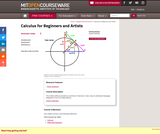Conditional Remix & Share Permitted
CC BY-NC-SA
Rating

This online textbook provides an overview of Calculus in clear, easy to understand language designed for the non-mathematician.

Subject:
Calculus
Material Type:
Full Course
Provider:
M.I.T.
Provider Set:
M.I.T. OpenCourseWare
Author:
Kleitman, Daniel
01/01/2005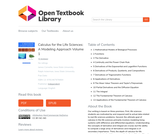Conditional Remix & Share Permitted
CC BY-NC-SA
Rating

Our writing is based on three premises. First, life sciences students are motivated by and respond well to actual data related to real life sciences problems. Second, the ultimate goal of calculus in the life sciences primarily involves modeling living systems with difference and differential equations. Understanding the concepts of derivative and integral are crucial, but the ability to compute a large array of derivatives and integrals is of secondary importance. Third, the depth of calculus for life sciences students should be comparable to that of the traditional physics and engineering calculus course; else life sciences students will be short changed and their faculty will advise them to take the 'best' (engineering) course.

Subject:
Calculus
Material Type:
Textbook
Provider:
University of Minnesota
Provider Set:
University of Minnesota Libraries Publishing
Author:
James L. Cornette
Ralph A. Ackerman
01/01/2012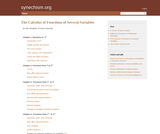Conditional Remix & Share Permitted
CC BY-NC-SA
Rating

This books covers the following topics: Geometry of Rn; functions from R to Rn; functions from Rn to R; functions from Rm to Rn.

Subject:
Calculus
Material Type:
Homework/Assignment
Textbook
Provider:
Furman University
Author:
Dan Sloughter
04/25/2019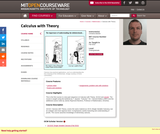Conditional Remix & Share Permitted
CC BY-NC-SA
Rating

Calculus with Theory, covers the same material as 18.01 (Single Variable Calculus), but at a deeper and more rigorous level. It emphasizes careful reasoning and understanding of proofs. The course assumes knowledge of elementary calculus.

Subject:
Calculus
Material Type:
Full Course
Provider:
M.I.T.
Provider Set:
M.I.T. OpenCourseWare
Author:
Breiner, Christine
01/01/2010Unrestricted Use
CC BY
Rating

Chapter 0 -- Review and Preview
Chapter 1 -- Functions, Graphs, Limits and Continuity
Chapter 2 -- The Derivative
Chapter 3 -- Derivatives and Graphs
Chapter 4 -- The Integral
Chapter 5 -- Applications of Definite Integrals
Chapter 6 -- Introduction to Differential Equations
Chapter 7 -- Inverse Trigonometric Functions
Chapter 8 -- Improper Integrals and Integration Techniques
Chapter 9 -- Polar, Parametric & Conics
Chapter 10 -- Infinite Series & Power Series
Chapter 11 -- Vectors, Lines and Planes in 3D
Chapter 12 -- Vector-Valued Functions
Chapter 13 -- Functions of Several Variables
Chapter 14 -- Double and Triple Integrals
Chapter 15 -- Vector Calculus

Subject:
Calculus
Material Type:
Textbook
Author:
Dale Hoffman
11/30/2020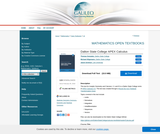Conditional Remix & Share Permitted
CC BY-NC-SA
Rating

This text for Analytic Geometry and Calculus I, II, and III is a Dalton State College remix of APEX Calculus 3.0.

Subject:
Mathematics
Calculus
Material Type:
Textbook
Author:
Jason Schmurr
Michael Hilgemann
Thomas Gonzalez
03/28/2019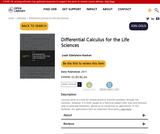Conditional Remix & Share Permitted
CC BY-NC-SA
Rating

Calculus arose as a tool for solving practical scientific problems through the centuries. However, it is often taught as a technical subject with rules and formulas (and occasionally theorems), devoid of its connection to applications. In this textbook, the applications form an important focal point, with emphasis on life sciences. This places the techniques and concepts into practical context, as well as motivating quantitative approaches to biology taught to undergraduates. While many of the examples have a biological flavour, the level of biology needed to understand those examples is kept at a minimum. The problems are motivated with enough detail to follow the assumptions, but are simplified for the purpose of pedagogy.

1 Power functions as building blocks
2 Average rates of change, average velocity and the secant line
3 Three faces of the derivative: geometric, analytic, and computational
4 Differentiation rules, simple antiderivatives and applications
5 Tangent lines, linear approximation, and Newton’s method
6 Sketching the graph of a function using calculus tools
7 Optimization
8 Introducing the chain rule
9 Chain rule applied to related rates and implicit differentiation
10 Exponential functions
11 Differential equations for exponential growth and decay
12 Solving differential equations
13 Qualitative methods for differential equations
14 Periodic and trigonometric functions
15 Cycles, periods, and rates of change

Subject:
Calculus
Material Type:
Textbook
Author:
Leah Edelstein-Keshet
11/30/2020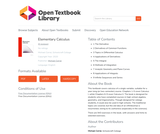Rating

This textbook covers calculus of a single variable, suitable for a year-long (or two-semester) course. Chapters 1-5 cover Calculus I, while Chapters 6-9 cover Calculus II. The book is designed for students who have completed courses in high-school algebra, geometry, and trigonometry. Though designed for college students, it could also be used in high schools. The traditional topics are covered, but the old idea of an infinitesimal is resurrected, owing to its usefulness (especially in the sciences).

There are 943 exercises in the book, with answers and hints to selected exercises.

1 The Derivative
2 Derivatives of Common Functions
3 Topics in Differential Calculus
4 Applications of Derivatives
5 The Integral
6 Methods of Integration
7 Analytic Geometry and Plane Curves
8 Applications of Integrals
9 Infinite Sequences and Series

Subject:
Calculus
Material Type:
Textbook
Author:
Michael Corral
01/20/2021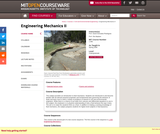Conditional Remix & Share Permitted
CC BY-NC-SA
Rating

This subject provides an introduction to fluid mechanics. Students are introduced to and become familiar with all relevant physical properties and fundamental laws governing the behavior of fluids and learn how to solve a variety of problems of interest to civil and environmental engineers. While there is a chance to put skills from Calculus and Differential Equations to use in this subject, the emphasis is on physical understanding of why a fluid behaves the way it does. The aim is to make the students think as a fluid. In addition to relating a working knowledge of fluid mechanics, the subject prepares students for higher-level subjects in fluid dynamics.

Subject:
Environmental Science
Calculus
Material Type:
Full Course
Provider:
M.I.T.
Provider Set:
M.I.T. OpenCourseWare
Author:
01/01/2006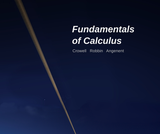Conditional Remix & Share Permitted
CC BY-SA
Rating

This open-source book by Crowell, Robbin, and Angenent is a spin-off of a previous open-source book by Robbin and Angenent. It covers the first semester of a freshman calculus course.

Subject:
Calculus
Material Type:
Textbook
Provider:
Light and Matter
Provider Set:
Light and Matter Books
Author:
Crowell
Robin and Angenent
04/25/2019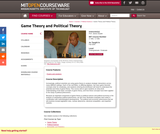Conditional Remix & Share Permitted
CC BY-NC-SA
Rating

Increasingly, political scientists are using game theory to analyze strategic interactions across many different settings. Each of the sub-fields, to differing degrees, has seen game theoretic concepts enter its vocabulary, and students entering the profession will need to understand the potential and limits of game theory. This course aims to give students an entry-level understanding of the basic concepts of game theory, and how these concepts have been applied to the study of political phenomena. Because an important component of game theory in political science and political economy is the analysis of substantive political phenomena, we will cover illustrative examples each week in combination with methodological developments. The political and economic phenomena that we will examine include legislative rules, nuclear deterrence, electoral competition, and imperfect markets.

Subject:
Calculus
Political Science
Material Type:
Full Course
Provider:
M.I.T.
Provider Set:
M.I.T. OpenCourseWare
Author:
Snyder, James
01/01/2004Conditional Remix & Share Permitted
CC BY-NC-SA
Rating

A rigorous introduction designed for mathematicians into perturbative quantum field theory, using the language of functional integrals. Basics of classical field theory. Free quantum theories. Feynman diagrams. Renormalization theory. Local operators. Operator product expansion. Renormalization group equation. The goal is to discuss, using mathematical language, a number of basic notions and results of QFT that are necessary to understand talks and papers in QFT and string theory.

Subject:
Calculus
Geometry
Material Type:
Full Course
Provider:
M.I.T.
Provider Set:
M.I.T. OpenCourseWare
Author:
Etingof, Pavel I.
01/01/2002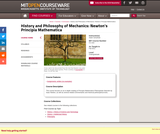Conditional Remix & Share Permitted
CC BY-NC-SA
Rating

This course focuses on an in-depth reading of Principia Mathematica Philosophiae Naturalis by Isaac Newton, as well as several related commentaries and historical philosophical texts.

Subject:
Arts and Humanities
Philosophy
Mathematics
Calculus
Material Type:
Full Course
Provider:
M.I.T.
Provider Set:
M.I.T. OpenCourseWare
Author:
01/01/2011Unrestricted Use
CC BY
Rating

This book is an approachable introduction to calculus with applications to biology and environmental science. For example, one application in the book is determining the volume of earth moved in the 1959 earthquake that created Quake Lake. Another application uses differential equations to model various biological examples, including moose and wolf populations at Isle Royale National Park, ranavirus in amphibians, and competing species of protozoa. The text focuses on intuitive understanding of concepts, but still covers most of the algebra and calculations common in a survey of calculus course.

Algebra Tips and Tricks: Part I
Algebra Tips and Tricks Part I (Combining Terms, Distributing, Functions, Graphing)
Homework for Algebra Tips and Tricks: Part I
Derivative Introduction
Ball Toss Project
Position to Velocity
Homework: Position to Velocity
Algebra Tips and Tricks Part II (Piecewise Defined Functions)
Limits
Homework: Limits
Algebra Tips and Tricks Part III (Factoring)
Algebraic Limits
Homework: Algebraic Limits
Instantaneous Velocity
Homework: Instantaneous Velocity
Algebra Tips and Tricks IV (Tips for dealing with fractions)
Definition of Derivative Examples
Project: Hard Definition of Derivative Problems
Homework: Examples of the Definition of the Derivative
Project: Killdeer Migration Speed
Rules for Derivatives
Algebra Tips and Tricks Part V (Exponents)
Power Rule
Homework: Power Rule
Algebra Tips and Tricks Part VI (Logarithms)
Exponentials, Logarithms, and Trig Functions
Homework: Exponents, Logs, Trig Functions
Product Rule
Homework: Product Rule
Quotient Rule
Homework: Quotient Rule
Chain Rule
Homework: Chain Rule
Multirule Derivatives
Homework: Multirule Derivatives
Anti-derivatives
Homework: Anti-derivatives
More Derivative Intuition
Derivatives and Graphs
Homework: Derivative Graphs
Second Derivatives and Interpreting the Derivative
Homework: Second Derivatives and Interpreting the Derivative
Optimization
Homework: Optimization
Derivatives in Space
Homework: Derivatives in Space
Differential Equations
Recurrance Relations
Homework: Recurrence Relations
Introduction to Differential Equations
Homework: Introduction to Differential Equations
Understanding Differential Equations
Homework: Understanding Differential Equations
Initial Value Problems
Homework: Initial Value Problems
Growth and Decay
Homework: Growth and Decay
Exploring Graphs of Differential Equations
Project: Modelling with Differential Equations
Intuition for Integration
Introduction to Integrals
Numeric Integration Techniques
Homework: Numeric Integration Techniques
Fundamental Theorem of Calculus
Homework: The Fundamental Theorem of Calculus
Project: Measuring Streamflow
Project: Quake Lake
Rules for Integration
Power, exponential, trig, and logarithm rules
Homework: Power, exponential, trig, and logarithmic rules
u-substitution
Homework: u-substitution
Integral Applications
Homework: Integral Applications
Integration by Parts
Homework: Integration by Parts
Acknowledgements

Subject:
Calculus
Material Type:
Textbook
Author:
Debbie Seacrest
Michele Anderson
Rebekah Levine
Tyler Seacrest
09/21/2021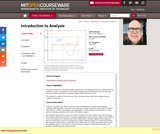Conditional Remix & Share Permitted
CC BY-NC-SA
Rating

Analysis I in its various versions covers fundamentals of mathematical analysis: continuity, differentiability, some form of the Riemann integral, sequences and series of numbers and functions, uniform convergence with applications to interchange of limit operations, some point-set topology, including some work in Euclidean n-space. MIT students may choose to take one of three versions of 18.100: Option A (18.100A) chooses less abstract definitions and proofs, and gives applications where possible. Option B (18.100B) is more demanding and for students with more mathematical maturity; it places more emphasis from the beginning on point-set topology and n-space, whereas Option A is concerned primarily with analysis on the real line, saving for the last weeks work in 2-space (the plane) and its point-set topology. Option C (18.100C) is a 15-unit variant of Option B, with further instruction and practice in written and oral communication.

Subject:
Calculus
Material Type:
Full Course
Provider:
M.I.T.
Provider Set:
M.I.T. OpenCourseWare
Author:
Arthur Mattuck
01/01/2012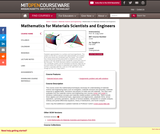Conditional Remix & Share Permitted
CC BY-NC-SA
Rating

This course covers the mathematical techniques necessary for understanding of materials science and engineering topics such as energetics, materials structure and symmetry, materials response to applied fields, mechanics and physics of solids and soft materials. The class uses examples from the materials science and engineering core courses (3.012 and 3.014) to introduce mathematical concepts and materials-related problem solving skills. Topics include linear algebra and orthonormal basis, eigenvalues and eigenvectors, quadratic forms, tensor operations, symmetry operations, calculus of several variables, introduction to complex analysis, ordinary and partial differential equations, theory of distributions, and fourier analysis. Users may find additional or updated materials at Professor Carter's 3.016 course Web site.

Subject:
Calculus
Material Type:
Full Course
Provider:
M.I.T.
Provider Set:
M.I.T. OpenCourseWare
Author:
Carter, W. Craig
01/01/2005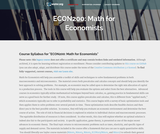Unrestricted Use
CC BY
Rating

A math based economics course designed to provide the skills needed to solve fundamental problems in both macroeconomics and microeconomics, by covering concepts in precalculus and calculus.

Subject:
Calculus
Economics
Material Type:
Full Course
Provider:
The Saylor Foundation
04/29/2019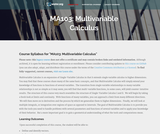Unrestricted Use
CC BY
Rating

Multivariable Calculus is an expansion of Single-Variable Calculus in that it extends single variable calculus to higher dimensions. This course begins with a fresh look at limits and continuity, moves to derivatives and the process of generalizing them to higher dimensions, and finally examines multiple integrals (integration over regions of space as opposed to intervals). Upon successful completion of this course, the student will be able to: Define and identify vectors; Define and compute dot and cross-products; Solve problems involving the geometry of lines, curves, planes, and surfaces in space; Define and compute velocity and acceleration in space; Define and solve Kepler's Second Law; Define and compute partial derivatives; Define and determine tangent planes and level curves; Define and compute least squares; Define and determine boundaries and infinity; Define and determine differentials and the directional derivative; Define and compute the gradient and the directional derivative; Define, determine, and apply Lagrange multipliers to solve problems; Define and compute partial differential equations; Define and evaluate double integrals; Use rectangular coordinates to solve problems in multivariable calculus; Use polar coordinates to solve problems in multivariable calculus; Use change of variables to evaluate integrals; Define and use vector fields and line integrals to solve problems in multivariable calculus; Define and verify conservative fields and path independence; Define and determine gradient fields and potential functions; Use Green's Theorem to evaluate and solve problems in multivariable calculus; Define flux; Define and evaluate triple integrals; Define and use rectangular coordinates in space; Define and use cylindrical coordinates; Define and use spherical coordinates; Define and correctly manipulate vector fields in space; Evaluate surface integrals and relate them to flux; Use the Divergence Theorem (Gauss' Theorem) to solve problems in multivariable calculus; Define and evaluate line integrals in space; Apply Stokes' Theorem to solve problems in multivariable calculus; Properly apply Maxwell's Equations to solve problems. (Mathematics 103)

Subject:
Calculus
Material Type:
Full Course
Provider:
The Saylor Foundation
04/29/2019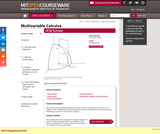Conditional Remix & Share Permitted
CC BY-NC-SA
Rating

This course covers differential, integral and vector calculus for functions of more than one variable. These mathematical tools and methods are used extensively in the physical sciences, engineering, economics and computer graphics.

Subject:
Calculus
Material Type:
Full Course
Provider:
M.I.T.
Provider Set:
M.I.T. OpenCourseWare
Author:
Auroux, Denis
01/01/2010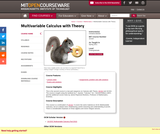Conditional Remix & Share Permitted
CC BY-NC-SA
Rating

This course is a continuation of 18.014. It covers the same material as 18.02 (Multivariable Calculus), but at a deeper level, emphasizing careful reasoning and understanding of proofs. There is considerable emphasis on linear algebra and vector integral calculus.

Subject:
Calculus
Material Type:
Full Course
Provider:
M.I.T.
Provider Set:
M.I.T. OpenCourseWare
Author:
Breiner, Christine
01/01/2011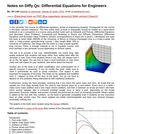Conditional Remix & Share Permitted
CC BY-NC-SA
Rating

A first one-semester course on differential equations aimed at engineers.

First order ODEs
Higher order linear ODEs
Systems of ODEs
Fourier series and PDEs
Eigenvalue problems
The Laplace transform
Power series methods
Nonlinear systems

Reviews available here: https://open.umn.edu/opentextbooks/textbooks/notes-on-diffy-qs-differential-equations-for-engineers

Subject:
Engineering
Calculus
Material Type:
Full Course
Textbook
Provider:
University of Illinois at Urbana-Champaign
Provider Set:
Individual Authors
Author:
Jiri Lebl
04/24/2019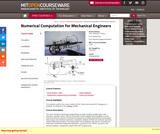Conditional Remix & Share Permitted
CC BY-NC-SA
Rating

This class introduces elementary programming concepts including variable types, data structures, and flow control. After an introduction to linear algebra and probability, it covers numerical methods relevant to mechanical engineering, including approximation (interpolation, least squares and statistical regression), integration, solution of linear and nonlinear equations, ordinary differential equations, and deterministic and probabilistic approaches. Examples are drawn from mechanical engineering disciplines, in particular from robotics, dynamics, and structural analysis. Assignments require MATLAB programming.

Subject:
Engineering
Information Science
Calculus
Statistics and Probability
Material Type:
Full Course
Provider:
M.I.T.
Provider Set:
M.I.T. OpenCourseWare
Author:
Anthony Patera
Daniel Frey
01/01/2012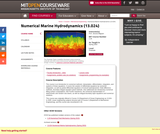Conditional Remix & Share Permitted
CC BY-NC-SA
Rating

Introduction to numerical methods: interpolation, differentiation, integration, systems of linear equations. Solution of differential equations by numerical integration, partial differential equations of inviscid hydrodynamics: finite difference methods, panel methods. Fast Fourier Transforms. Numerical representation of sea waves. Computation of the motions of ships in waves. Integral boundary layer equations and numerical solutions.

Subject:
Calculus
Material Type:
Full Course
Provider:
M.I.T.
Provider Set:
M.I.T. OpenCourseWare
Author:
Prof. Jerome Milgram
01/01/2003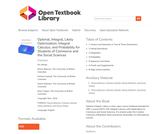Conditional Remix & Share Permitted
CC BY-NC-SA
Rating

Optimal, Integral, Likely is a free, open-source textbook intended for UBC’s course MATH 105: Integral Calculus with Applications to Commerce and Social Sciences. It is shared under the Creative Commons Attribution-NonCommercial-ShareAlike 4.0 International License.

1 Vectors and Geometry in Two & Three Dimensions
2 Partial Derivatives
3 Integration
4 Probability
5 Sequence and Series
A Proofs and Supplements
B High school material

Subject:
Calculus
Statistics and Probability
Social Science
Material Type:
Textbook
Author:
Bruno Belevan
Elyse Yeager
Nisha Malhotra
Parham Hamidi
09/21/2021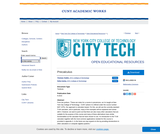Conditional Remix & Share Permitted
CC BY-NC-SA
Rating

From the preface, "These are notes for a course in precalculus, as it is taught at New York City College of Technology - CUNY (where it is offered under the course number MAT 1375). Our approach is calculator based. For this, we will use the currently standard TI-84 calculator, and in particular, many of the examples will be explained and solved with it. However, we want to point out that there are also many other calculators that are suitable for the purpose of this course and many of these alternatives have similar functionalities as the calculator that we have chosen to use. An introduction to the TI-84 calculator together with the most common applications needed for this course is provided in appendix A. In the future we may expand on this by providing introductions to other calculators or computer algebra systems."

I Functions and graphs

1 The absolute value
2 Lines and functions
3 Functions by formulas and graphs
4 Introduction to the TI-84
5 Basic functions and transformations
6 Operations on functions
7 The inverse of a function
II Polynomials and rational functions

8 Dividing polynomials
9 Graphing polynomials
10 Roots of polynomials
11 Rational functions
12 Polynomial and rational inequalities
III Exponential and logarithmic functions

13 Exponential and logarithmic functions
14 Properties of exp and log
15 Applications of exp and log
16 Half-life and compound interest
IV Trigonometric functions

17 Trigonometric functions
18 Addition of angles and multiple angles
19 Inverse trigonometric functions
20 Trigonometric equations
V Complex numbers, sequences, and the binomial theorem

21 Complex numbers
22 Vectors in the plane
23 Sequences and series
24 The geometric series
25 The binomial theorem

Subject:
Calculus
Material Type:
Textbook
Provider:
Provider Set:
New York City College of Technology
Author:
Holly Carley
09/01/2015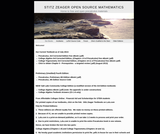Conditional Remix & Share Permitted
CC BY-NC-SA
Rating

Open source precalculus textbook.

able of Contents
1 Relations and Functions
3 Polynomial Functions
4 Rational Functions
5 Further Topics in Functions
6 Exponential and Logarithmic Functions
7 Hooked on Conics
8 Systems of Equations and Matrices
9 Sequences and the Binomial Theorem
10 Foundations of Trigonometry
11 Applications of Trigonometry

Access also available here: http://www.stitz-zeager.com/index.html

Subject:
Calculus
Material Type:
Textbook
Provider:
Stitz-Zeager Open Source Mathematics
Author:
Carl Stitz
Jeff Zeager
07/15/2011Some Rights Reserved
Rating

Precalculus 1 & 2 / Trigonometry provides a study of functions and their graphs, including polynomial, rational, exponential, and logarithmic functions. Additionally, right-triangle trigonometry, trigonometric functions and their applications are covered.

Subject:
Calculus
Trigonometry
Material Type:
Full Course
Textbook
Provider:
Lumen Learning
Provider Set:
Candela Courseware
Author:
David Lippman
Melonie Rasmussen
04/25/2019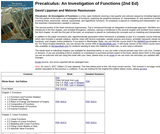Conditional Remix & Share Permitted
CC BY-SA
Rating

Precalculus: An Investigation of Functions is a free, open textbook covering a two-quarter pre-calculus sequence including trigonometry. The first portion of the book is an investigation of functions, exploring the graphical behavior of, interpretation of, and solutions to problems involving linear, polynomial, rational, exponential, and logarithmic functions. An emphasis is placed on modeling and interpretation, as well as the important characteristics needed in calculus.

Access also available here: http://www.opentextbookstore.com/precalc/

Chapter 1: Functions
Chapter 2: Linear Functions
Chapter 3: Polynomial and Rational Functions
Chapter 4: Exponential and Logarithmic Functions
Chapter 5: Trigonometric Functions of Angles
Chapter 6: Periodic Functions
Chapter 7: Trigonometric Equations and Identities
Chapter 8: Further Applications of Trigonometry

Subject:
Calculus
Material Type:
Textbook
Provider:
Open Textbook Network
Provider Set:
Pierce College
Author:
David Lippman
Melonie Rasmussen
01/01/2012Unrestricted Use
CC BY
Rating

This course is oriented toward US high school students. Mathematics comes together in this course. You enter precalculus with an abundant array of experience in mathematics, and this course offers an opportunity to make connections among the big ideas you encountered earlier. It also assists you in developing fluency with the tools used in learning calculus.

Subject:
Calculus
Functions
Material Type:
Full Course
Provider:
The Saylor Foundation
04/29/2019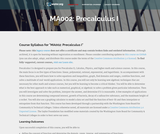Unrestricted Use
CC BY
Rating

Precalculus I is designed to prepare you for Precalculus II, Calculus, Physics, and higher math and science courses. In this course, the main focus is on five types of functions: linear, polynomial, rational, exponential, and logarithmic. In accompaniment with these functions, you will learn how to solve equations and inequalities, graph, find domains and ranges, combine functions, and solve a multitude of real-world applications.

Subject:
Calculus
Material Type:
Full Course
Provider:
The Saylor Foundation
04/29/2019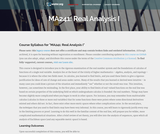Unrestricted Use
CC BY
Rating

This course is designed to introduce the student to the rigorous examination of the real number system and the foundations of calculus. Analysis lies at the heart of the trinity of higher mathematics algebra, analysis, and topology because it is where the other two fields meet. Upon successful completion of this course, the student will be able to: Use set notation and quantifiers correctly in mathematical statements and proofs; Use proof by induction or contradiction when appropriate; Define the rational numbers, the natural numbers, and the real numbers, and understand their relationship to one another; Define the well-ordering principle the completeness/supremum property of the real line, and the Archimedean property; Prove the existence of irrational numbers; Define supremum and infimum; Correctly and fluently manipulate expressions with absolute value and state the triangle inequality; Define and identify injective, surjective, and bijective mappings; Name the various cardinalities of sets and identify the cardinality of a given set; Define Euclidean space and vector space and show that Euclidean space is a vector space; Define the complex numbers and manipulate them algebraically; Write equations for lines and planes in Euclidean space; Define a normed linear space, a norm, and an inner product; Define metric spaces, open sets; define open, closed, and bounded sets; define cluster points; define density; Define convergence of sequences and prove or disprove the convergence of given sequences; Prove and use properties of limits; Prove standard results about closures, intersections, and unions of open and closed sets; Define compactness using both open covers and sequences; State and prove the Heine-Borel Theorem; State the Bolzano-Weierstrass Theorem; State and use the Cantor Finite Intersection Property; Define Cauchy sequence and prove that specific sequences are Cauchy; Define completeness and prove that Euclidean space with the standard metric is complete; Show that convergent sequences are Cauchy; Define limit superior and limit inferior; Define convergence of series using the Cauchy criterion and use the comparison, ratio, and root tests to show convergence of series; Define continuity and state, prove, and use properties of limits of continuous functions, including the fact that continuous functions attain extreme values on compact sets; Define divergence of functions to infinity and use properties of infinite limits; State and prove the intermediate value property; Define uniform continuity and show that given functions are or are not uniformly continuous; Give standard examples of discontinuous functions, such as the Dirichlet function; Define connectedness and identify connected and disconnected sets Construct the Cantor ternary set and state its properties; Distinguish between pointwise and uniform convergence; Prove that if a sequence of continuous functions converges uniformly, their limit is also continuous; Define derivatives of real- and extended-real-valued functions; Compute derivatives using the limit definition and prove basic properties of derivatives; State the Mean Value Theorem and use it in proofs; Construct the Riemann Integral and state its properties; State the Fundamental Theorem of Calculus and use it in proofs; Define pointwise and uniform convergence of series of functions; Use the Weierstrass M-Test to check for uniform convergence of series; Construct Taylor Series and state Taylor's Theorem; Identify necessary and sufficient conditions for term-by-term differentiation of power series. (Mathematics 241)

Subject:
Calculus
Material Type:
Full Course
Provider:
The Saylor Foundation
04/29/2019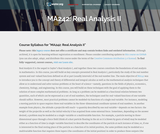Unrestricted Use
CC BY
Rating

Further study of real numbers, their real-valued functions, and integration and differentiation of these functions through the analysis of Manifolds.

Subject:
Calculus
Material Type:
Full Course
Provider:
The Saylor Foundation